# Discrete Mathematics Lecture 22 Recursion First of all

• Slides: 47Discrete Mathematics Lecture # 22 RecursionFirst of all instead of giving the definition of Recursion we give you an example, you already know the Set of Odd numbers. Here we give the new definition of the same set that is the set of Odd numbers.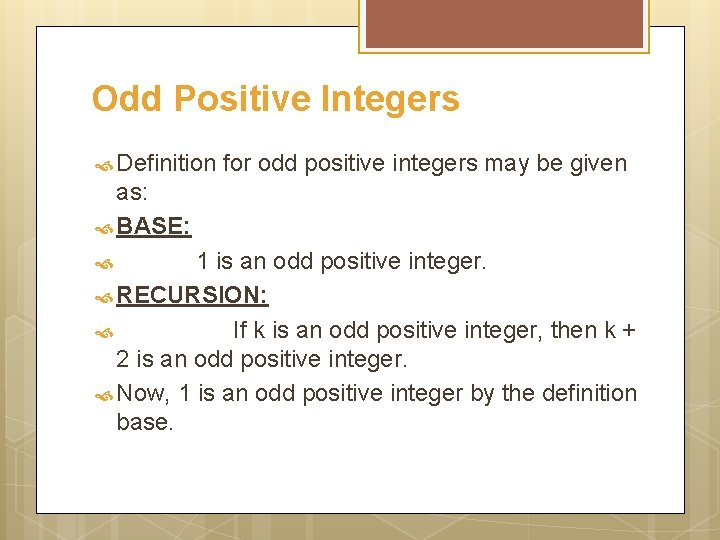Odd Positive Integers Definition for odd positive integers may be given as: BASE: 1 is an odd positive integer. RECURSION: If k is an odd positive integer, then k + 2 is an odd positive integer. Now, 1 is an odd positive integer by the definition base.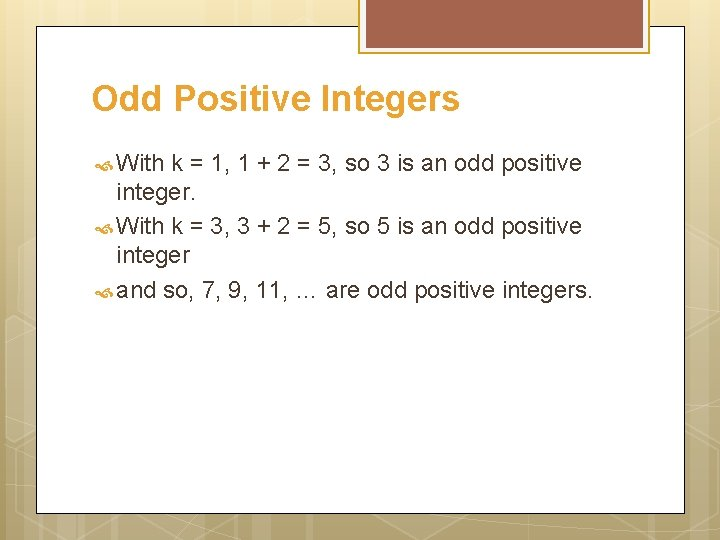Odd Positive Integers With k = 1, 1 + 2 = 3, so 3 is an odd positive integer. With k = 3, 3 + 2 = 5, so 5 is an odd positive integer and so, 7, 9, 11, … are odd positive integers.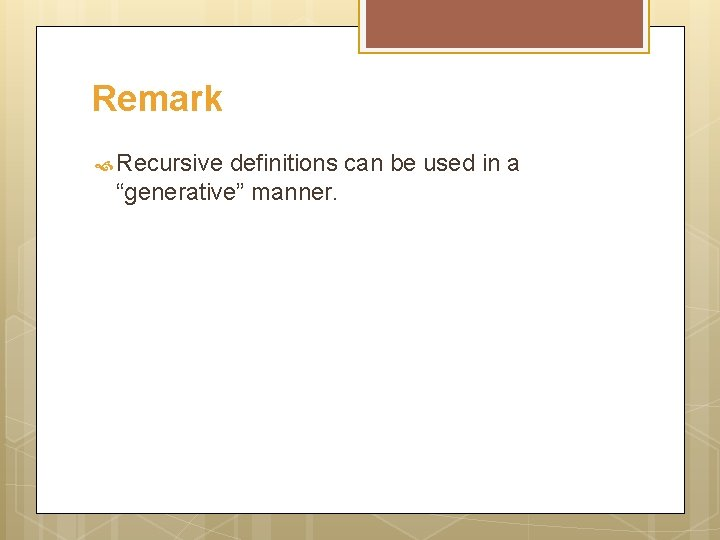Remark Recursive definitions can be used in a “generative” manner.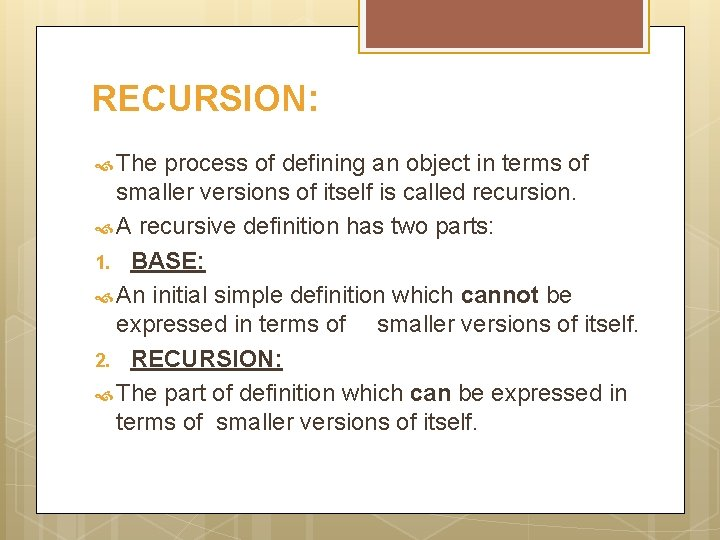RECURSION: The process of defining an object in terms of smaller versions of itself is called recursion. A recursive definition has two parts: 1. BASE: An initial simple definition which cannot be expressed in terms of smaller versions of itself. 2. RECURSION: The part of definition which can be expressed in terms of smaller versions of itself.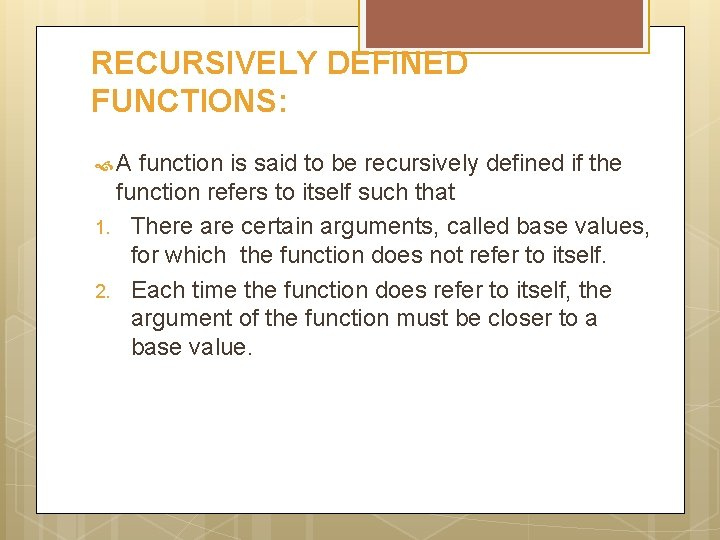RECURSIVELY DEFINED FUNCTIONS: A function is said to be recursively defined if the function refers to itself such that 1. There are certain arguments, called base values, for which the function does not refer to itself. 2. Each time the function does refer to itself, the argument of the function must be closer to a base value.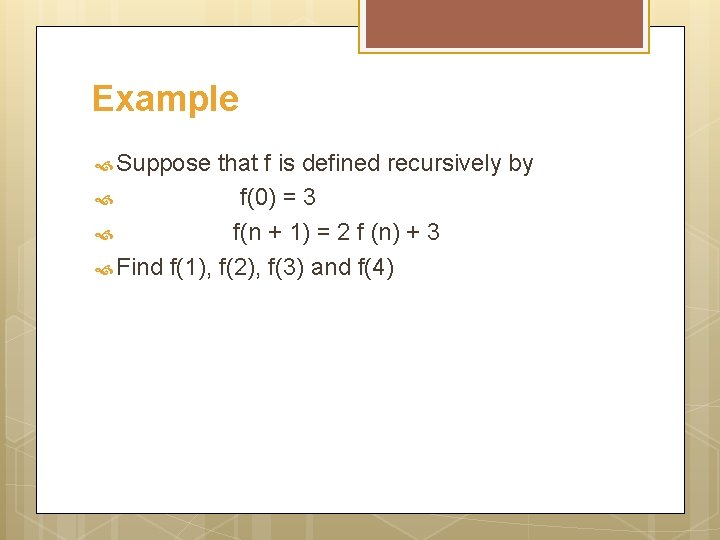Example Suppose that f is defined recursively by f(0) = 3 f(n + 1) = 2 f (n) + 3 Find f(1), f(2), f(3) and f(4)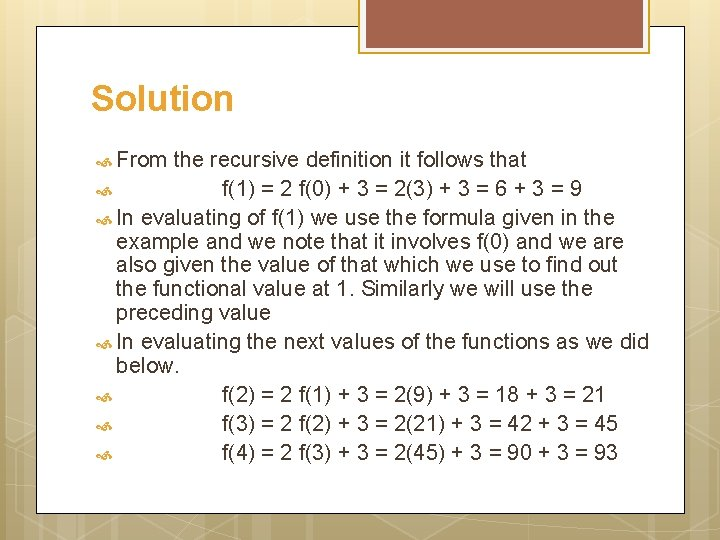Solution From the recursive definition it follows that f(1) = 2 f(0) + 3 = 2(3) + 3 = 6 + 3 = 9 In evaluating of f(1) we use the formula given in the example and we note that it involves f(0) and we are also given the value of that which we use to find out the functional value at 1. Similarly we will use the preceding value In evaluating the next values of the functions as we did below. f(2) = 2 f(1) + 3 = 2(9) + 3 = 18 + 3 = 21 f(3) = 2 f(2) + 3 = 2(21) + 3 = 42 + 3 = 45 f(4) = 2 f(3) + 3 = 2(45) + 3 = 90 + 3 = 93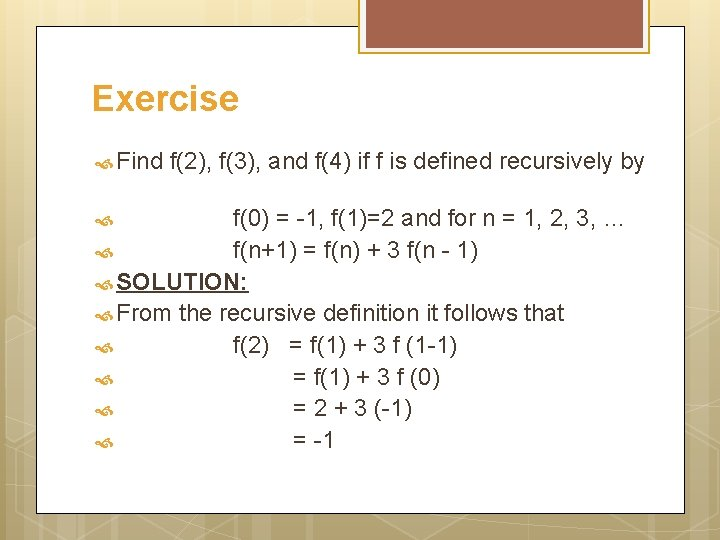Exercise Find f(2), f(3), and f(4) if f is defined recursively by f(0) = -1, f(1)=2 and for n = 1, 2, 3, … f(n+1) = f(n) + 3 f(n - 1) SOLUTION: From the recursive definition it follows that f(2) = f(1) + 3 f (1 -1) = f(1) + 3 f (0) = 2 + 3 (-1) = -1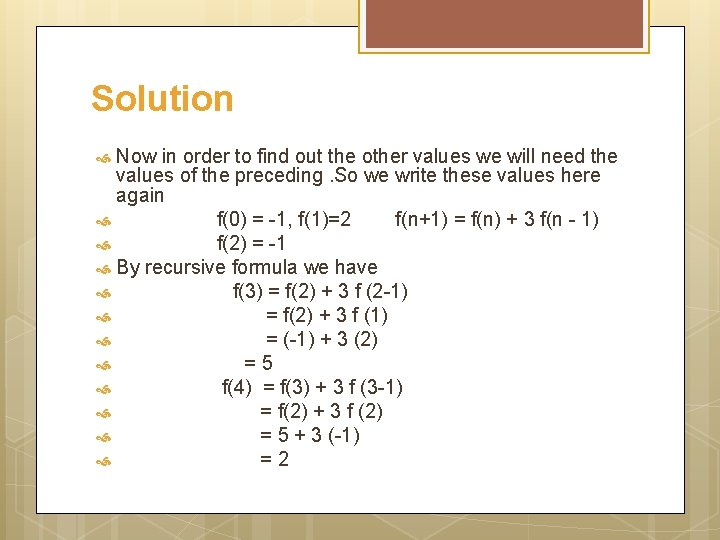Solution Now in order to find out the other values we will need the values of the preceding. So we write these values here again f(0) = -1, f(1)=2 f(n+1) = f(n) + 3 f(n - 1) f(2) = -1 By recursive formula we have f(3) = f(2) + 3 f (2 -1) = f(2) + 3 f (1) = (-1) + 3 (2) = 5 f(4) = f(3) + 3 f (3 -1) = f(2) + 3 f (2) = 5 + 3 (-1) = 2Example For each positive integer n, the factorial of n denoted n! is defined to be the product of all the integers from 1 to n: n! = n·(n - 1)·(n - 2) · · · 3 · 2 · 1 Zero factorial is defined to be 1 0! = 1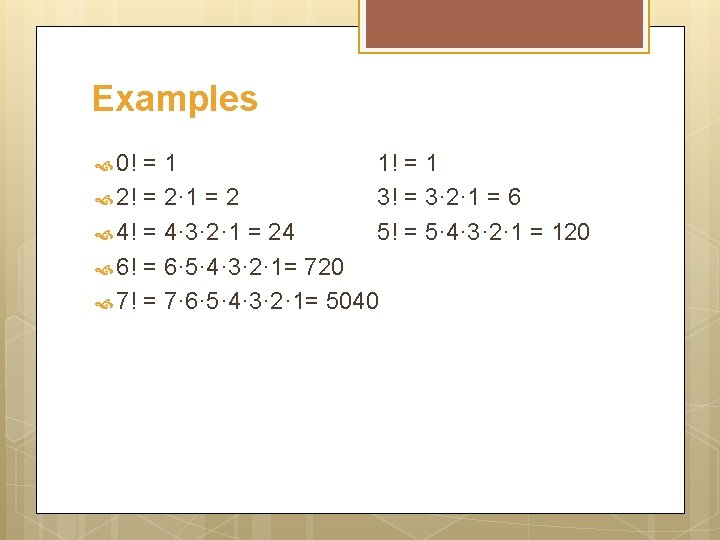Examples 0! = 1 2! = 2· 1 = 2 4! = 4· 3· 2· 1 = 24 1! = 1 3! = 3· 2· 1 = 6 5! = 5· 4· 3· 2· 1 = 120 6! = 6· 5· 4· 3· 2· 1= 720 7! = 7· 6· 5· 4· 3· 2· 1= 5040Remark 5! = 5 · 4 · 3 · 2 · 1 = 5 ·(4 · 3 · 2 · 1) = 5 · 4! In general, n! = n(n-1)! for each positive integer n.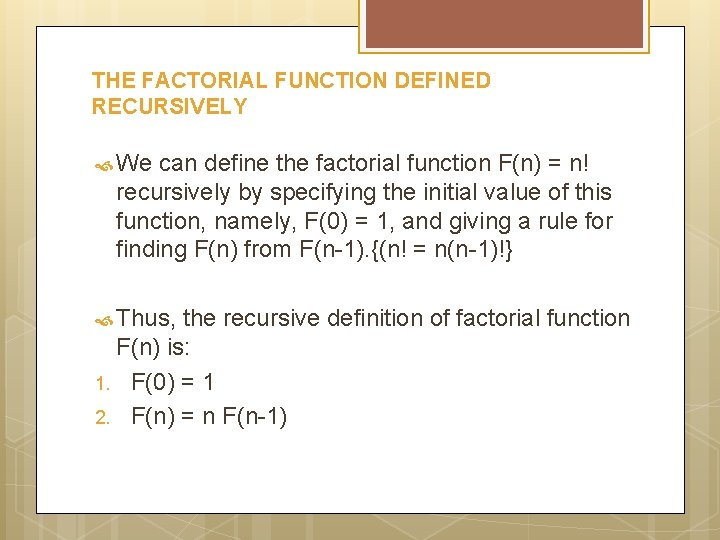THE FACTORIAL FUNCTION DEFINED RECURSIVELY We can define the factorial function F(n) = n! recursively by specifying the initial value of this function, namely, F(0) = 1, and giving a rule for finding F(n) from F(n-1). {(n! = n(n-1)!} Thus, the recursive definition of factorial function F(n) is: 1. F(0) = 1 2. F(n) = n F(n-1)Exercise Let S be the function such that S(n) is the sum of the first n positive integers. Give a recursive definition of S(n). SOLUTION: The initial value of this function may be specified as S(0) = 0 Since S(n) = n + (n - 1) + (n - 2) + … + 3 + 2 + 1 = n + [(n - 1) + (n - 2) + … + 3 + 2 + 1] = n + S(n-1) which defines the recursive step.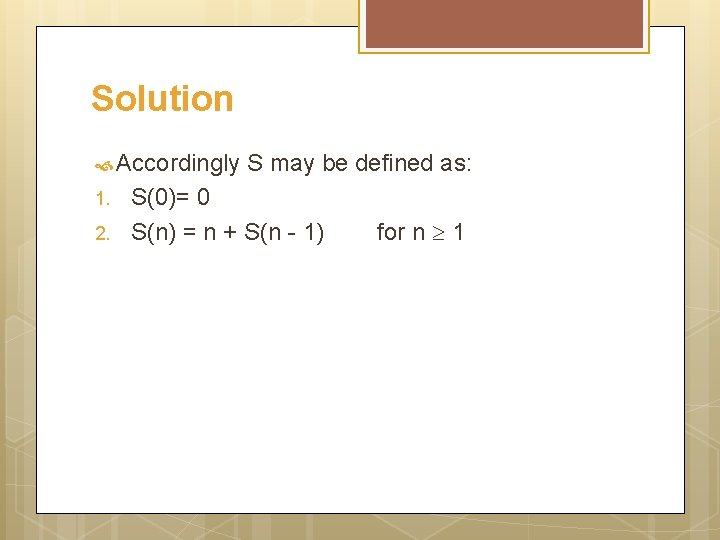Solution Accordingly S may be defined as: 1. 2. S(0)= 0 S(n) = n + S(n - 1) for n 1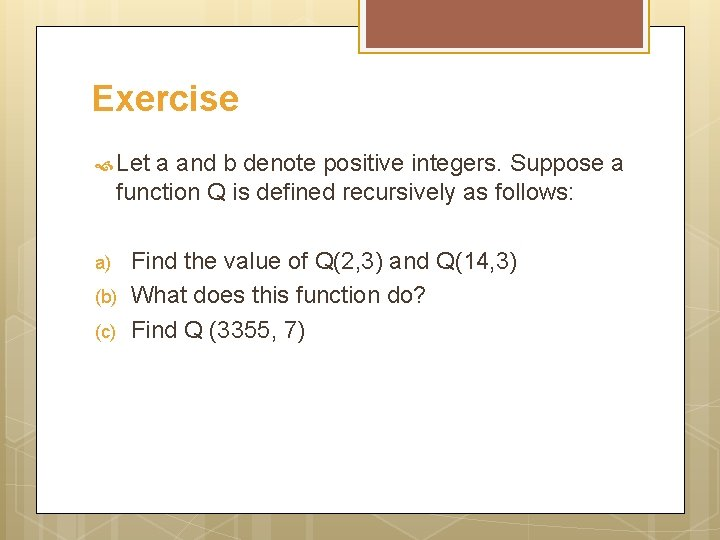Exercise Let a and b denote positive integers. Suppose a function Q is defined recursively as follows: a) (b) (c) Find the value of Q(2, 3) and Q(14, 3) What does this function do? Find Q (3355, 7)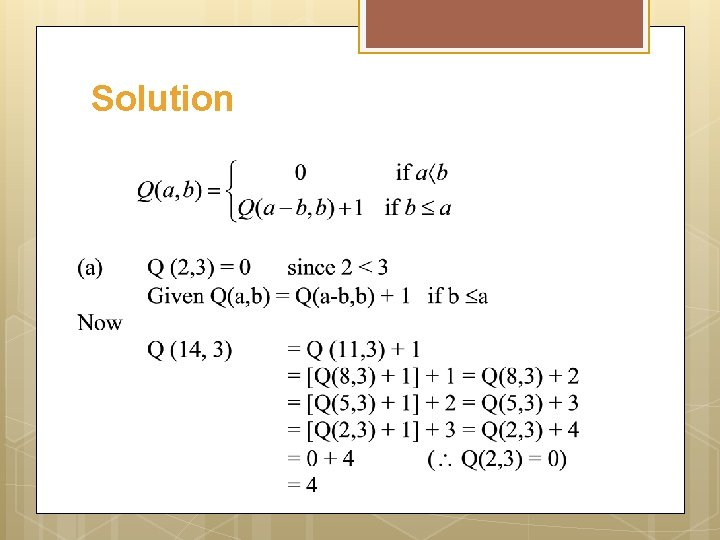SolutionSolutionTHE FIBONACCI SEQUENCE The Fibonacci sequence is defined as follows. F 0 = 1, F 1 = 1 Fk = Fk-1 + Fk-2 for all integers k 2 F 2 = F 1 + F 0 = 1 + 1 = 2 F 3 = F 2 + F 1 = 2 + 1 = 3 F 4 = F 3 + F 2 = 3 + 2 = 5 F 5 = F 4 + F 3 = 5 + 3 = 8 . . .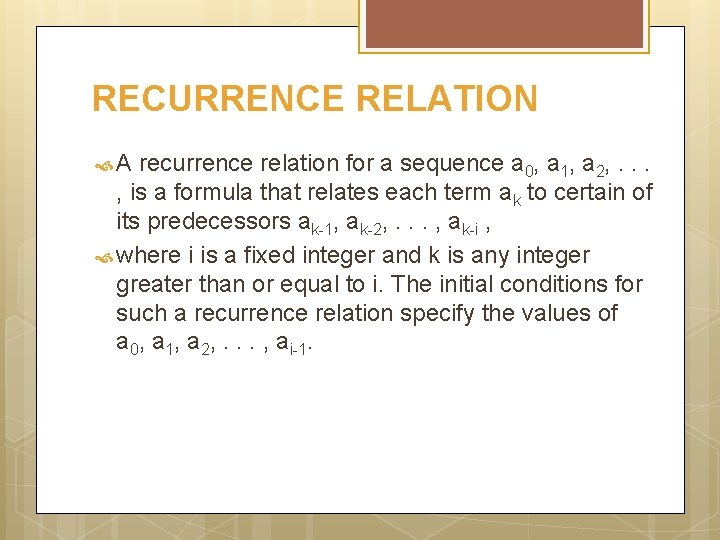RECURRENCE RELATION A recurrence relation for a sequence a 0, a 1, a 2, . . . , is a formula that relates each term ak to certain of its predecessors ak-1, ak-2, . . . , ak-i , where i is a fixed integer and k is any integer greater than or equal to i. The initial conditions for such a recurrence relation specify the values of a 0, a 1, a 2, . . . , ai-1.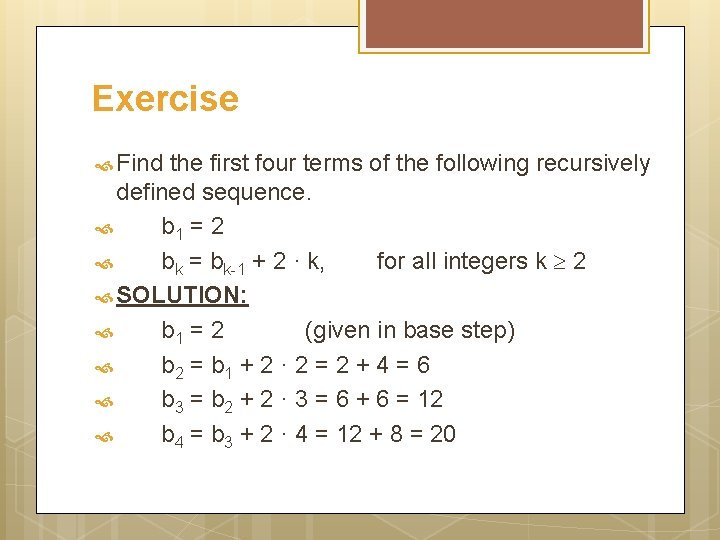Exercise Find the first four terms of the following recursively defined sequence. b 1 = 2 bk = bk-1 + 2 · k, for all integers k 2 SOLUTION: b 1 = 2 (given in base step) b 2 = b 1 + 2 · 2 = 2 + 4 = 6 b 3 = b 2 + 2 · 3 = 6 + 6 = 12 b 4 = b 3 + 2 · 4 = 12 + 8 = 20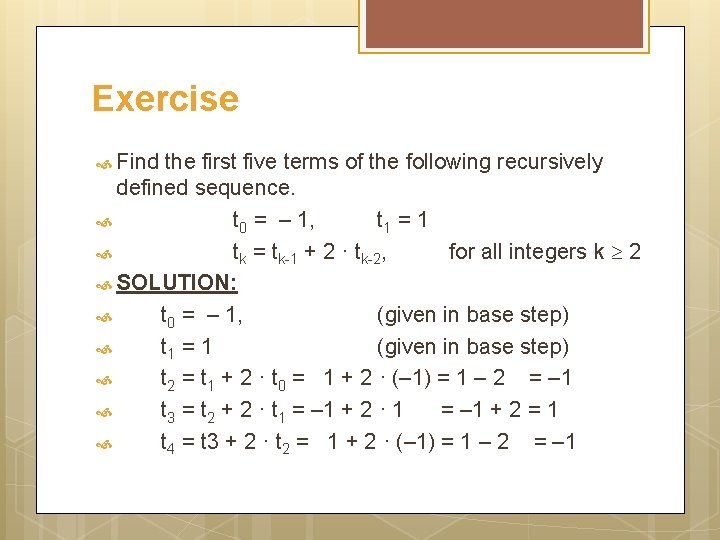Exercise Find the first five terms of the following recursively defined sequence. t 0 = – 1, t 1 = 1 tk = tk-1 + 2 · tk-2, for all integers k 2 SOLUTION: t 0 = – 1, (given in base step) t 1 = 1 (given in base step) t 2 = t 1 + 2 · t 0 = 1 + 2 · (– 1) = 1 – 2 = – 1 t 3 = t 2 + 2 · t 1 = – 1 + 2 · 1 = – 1 + 2 = 1 t 4 = t 3 + 2 · t 2 = 1 + 2 · (– 1) = 1 – 2 = – 1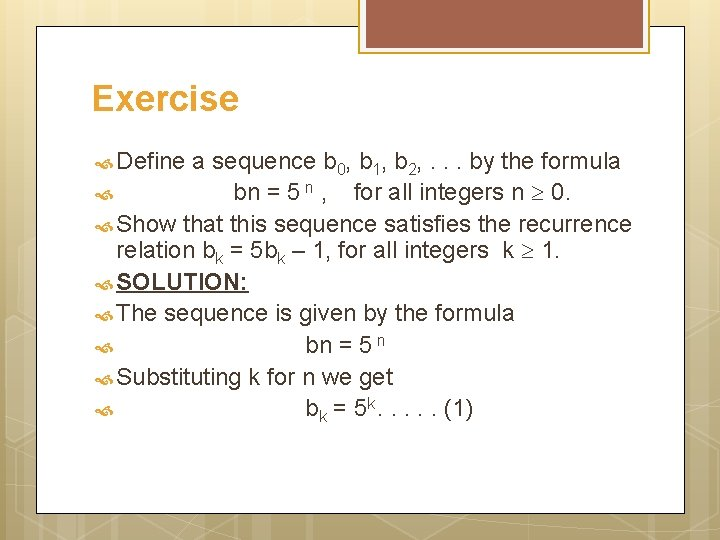Exercise Define a sequence b 0, b 1, b 2, . . . by the formula bn = 5 n , for all integers n 0. Show that this sequence satisfies the recurrence relation bk = 5 bk – 1, for all integers k 1. SOLUTION: The sequence is given by the formula bn = 5 n Substituting k for n we get bk = 5 k. . . (1)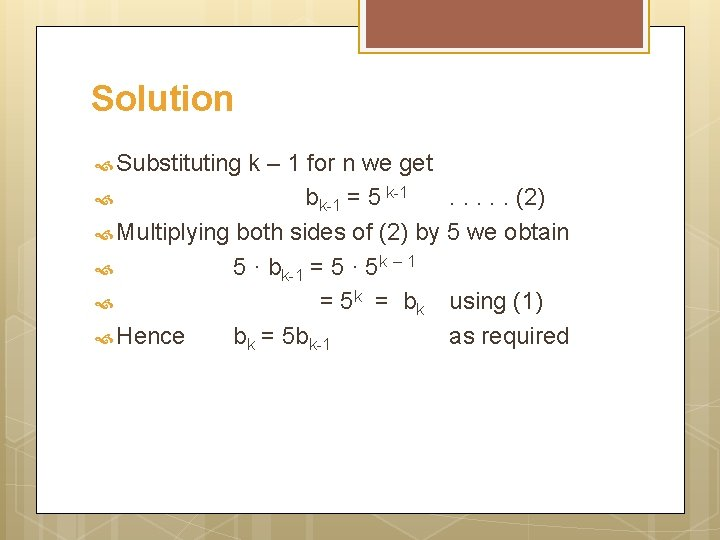Solution Substituting k – 1 for n we get bk-1 = 5 k-1. . . (2) Multiplying both sides of (2) by 5 we obtain 5 · bk-1 = 5 · 5 k – 1 = 5 k = bk using (1) Hence bk = 5 bk-1 as required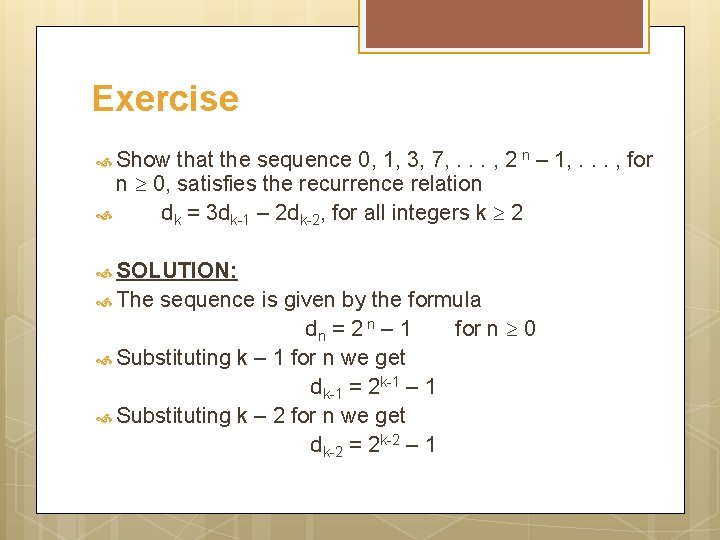Exercise Show that the sequence 0, 1, 3, 7, . . . , 2 n – 1, . . . , for n 0, satisfies the recurrence relation dk = 3 dk-1 – 2 dk-2, for all integers k 2 SOLUTION: The sequence is given by the formula dn = 2 n – 1 for n 0 Substituting k – 1 for n we get dk-1 = 2 k-1 – 1 Substituting k – 2 for n we get dk-2 = 2 k-2 – 1Solution We want to prove that dk = 3 dk-1 – 2 dk-2 R. H. S. = 3(2 k – 1) – 2(2 k – 2 – 1) = 3 · 2 k – 1 – 3 – 2 · 2 k – 2 + 2 = 3 · 2 k – 1 – 1 = (3 – 1) · 2 k – 1 = 2 · 2 k – 1 = 2 k – 1 = dk = L. H. S.THE TOWER OF HANOI The puzzle was invented by a French Mathematician Adouard Lucas in 1883. It is well known to students of Computer Science since it appears in virtually any introductory text on data structures or algorithms. There are three poles on first of which are stacked a number of disks that decrease in size as they rise from the base. The goal is to transfer all the disks one by one from the first pole to one of the others, but they must never place a larger disk on top of a smaller one.THE TOWER OF HANOI Let mn be the minimum number of moves needed to move a tower of n disks from one pole to another. Then mn can be obtained recursively as follows. m 1 = 1 mk = 2 mk-1 + 1 m 2 = 2 · m 1 + 1 = 2 · 1 + 1 = 3 m 3 = 2 · m 2 + 1 = 2 · 3 + 1 = 7 m 4 = 2 · m 3 + 1 = 2 · 7 + 1 = 15 m 5 = 2 · m 4 + 1 = 2 · 15 + 1 = 31 m 6 = 2 · m 5 + 1 = 2 · 31 + 1 = 63 Note that mn = 2 n – 1 m 64 = 264 – 1 584. 5 billion yearsUSE OF RECURSION At first recursion may seem hard or impossible, may be magical at best. However, recursion often provides elegant, short algorithmic solutions to many problems in computer science and mathematics. Examples where recursion is often used math functions number sequences data structure definitions data structure manipulations language definitions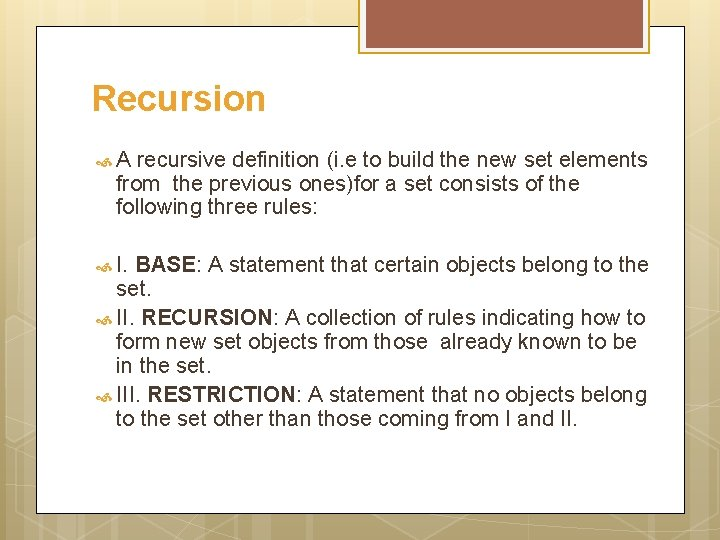Recursion A recursive definition (i. e to build the new set elements from the previous ones)for a set consists of the following three rules: I. BASE: A statement that certain objects belong to the set. II. RECURSION: A collection of rules indicating how to form new set objects from those already known to be in the set. III. RESTRICTION: A statement that no objects belong to the set other than those coming from I and II.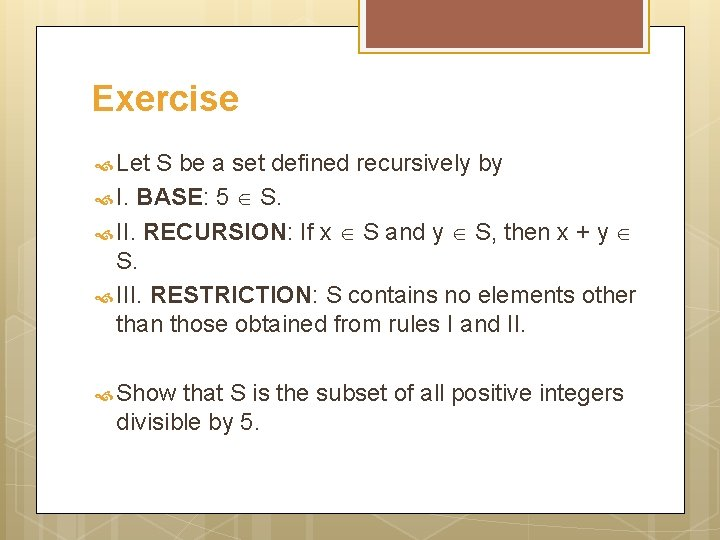Exercise Let S be a set defined recursively by I. BASE: 5 S. II. RECURSION: If x S and y S, then x + y S. III. RESTRICTION: S contains no elements other than those obtained from rules I and II. Show that S is the subset of all positive integers divisible by 5.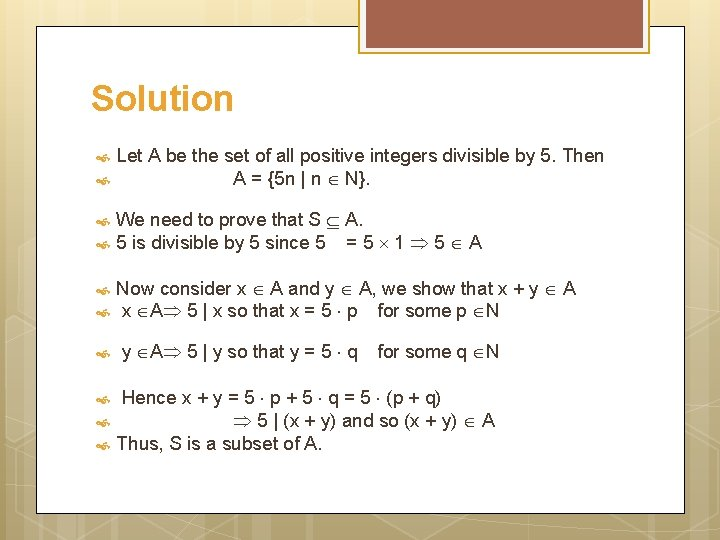Solution Let A be the set of all positive integers divisible by 5. Then A = {5 n | n N}. We need to prove that S A. 5 is divisible by 5 since 5 = 5 1 5 A Now consider x A and y A, we show that x + y A x A 5 | x so that x = 5 p for some p N y A 5 | y so that y = 5 q for some q N Hence x + y = 5 p + 5 q = 5 (p + q) 5 | (x + y) and so (x + y) A Thus, S is a subset of A.RECURSIVE DEFINITION OF BOOLEAN EXPRESSIONS I. BASE: Each symbol of the alphabet is a Boolean expression. II. RECURSION: If P and Q are Boolean Expressions, then so are (a) (P Q) (b) (P Q) and (c) ~ P. III RESTRICTION: There are no Boolean expressions over the alphabet other than those obtained from I and II.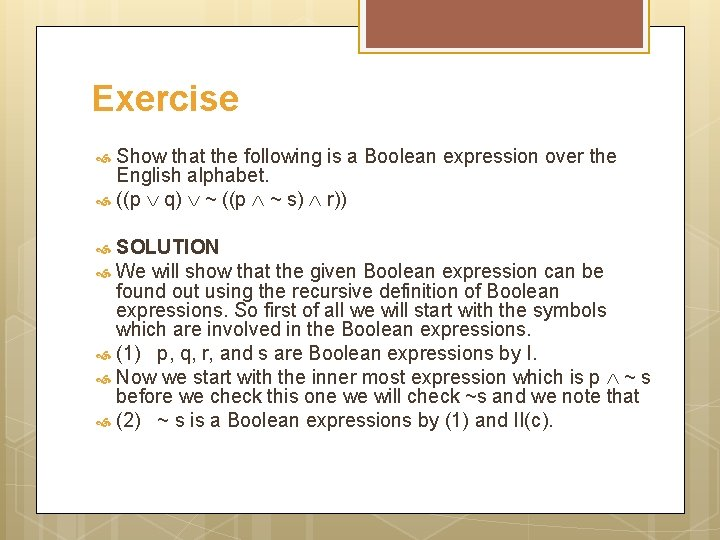Exercise Show that the following is a Boolean expression over the English alphabet. ((p q) ~ ((p ~ s) r)) SOLUTION We will show that the given Boolean expression can be found out using the recursive definition of Boolean expressions. So first of all we will start with the symbols which are involved in the Boolean expressions. (1) p, q, r, and s are Boolean expressions by I. Now we start with the inner most expression which is p ~ s before we check this one we will check ~s and we note that (2) ~ s is a Boolean expressions by (1) and II(c).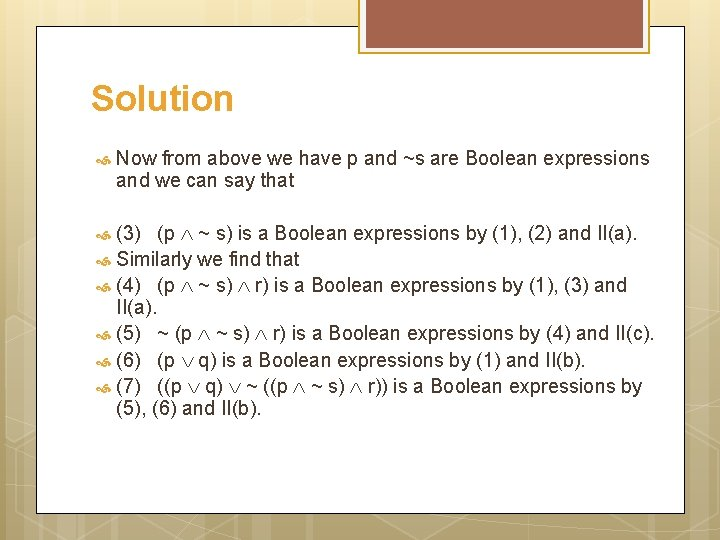Solution Now from above we have p and ~s are Boolean expressions and we can say that (3) (p ~ s) is a Boolean expressions by (1), (2) and II(a). Similarly we find that (4) (p ~ s) r) is a Boolean expressions by (1), (3) and II(a). (5) ~ (p ~ s) r) is a Boolean expressions by (4) and II(c). (6) (p q) is a Boolean expressions by (1) and II(b). (7) ((p q) ~ ((p ~ s) r)) is a Boolean expressions by (5), (6) and II(b).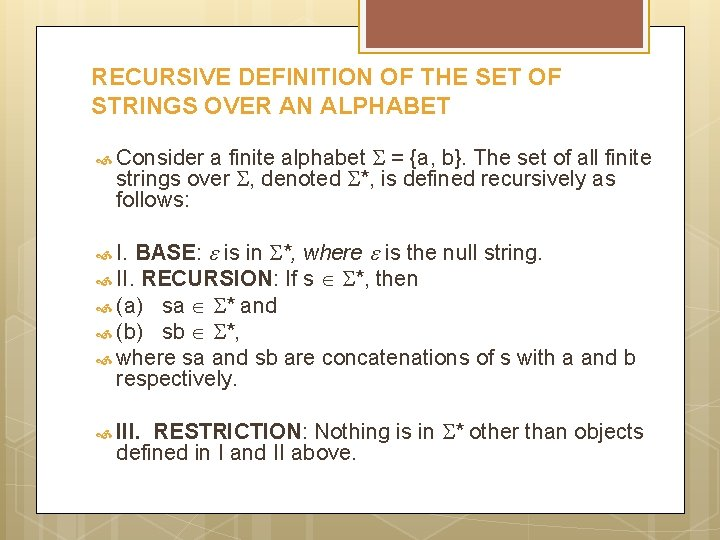RECURSIVE DEFINITION OF THE SET OF STRINGS OVER AN ALPHABET Consider a finite alphabet = {a, b}. The set of all finite strings over , denoted *, is defined recursively as follows: I. BASE: is in *, where is the null string. II. RECURSION: If s *, then (a) sa * and (b) sb *, where sa and sb are concatenations of s with a and b respectively. III. RESTRICTION: Nothing is in * other than objects defined in I and II above.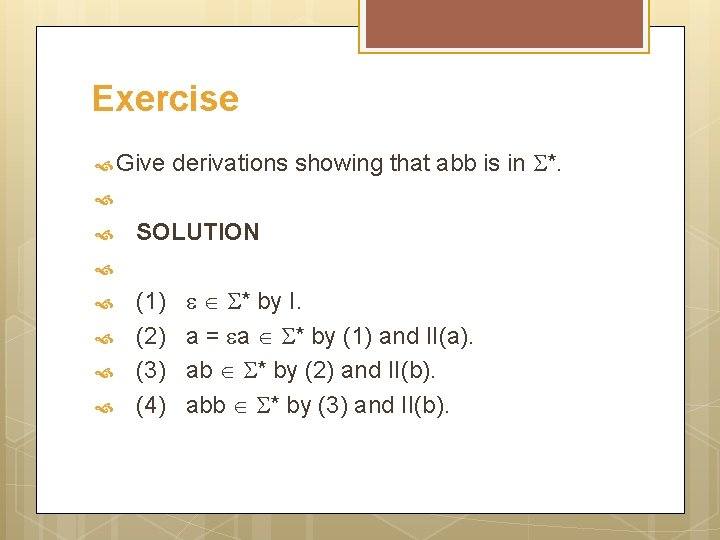Exercise Give derivations showing that abb is in *. SOLUTION (1) * by I. (2) a = a * by (1) and II(a). (3) ab * by (2) and II(b). (4) abb * by (3) and II(b).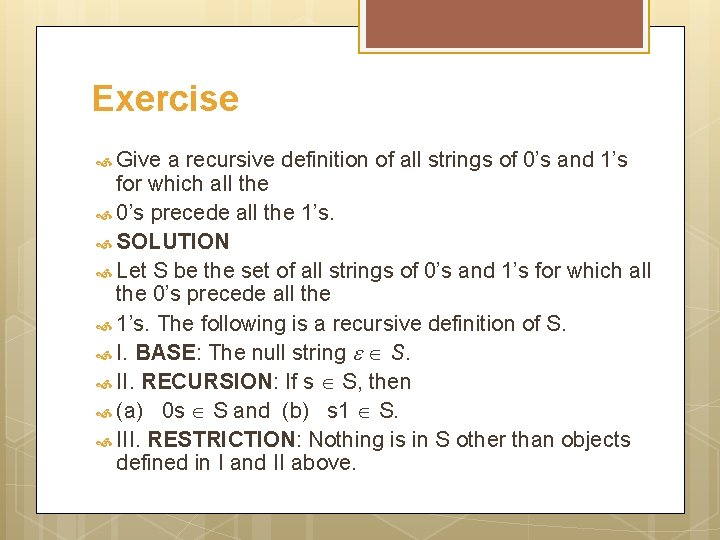Exercise Give a recursive definition of all strings of 0’s and 1’s for which all the 0’s precede all the 1’s. SOLUTION Let S be the set of all strings of 0’s and 1’s for which all the 0’s precede all the 1’s. The following is a recursive definition of S. I. BASE: The null string S. II. RECURSION: If s S, then (a) 0 s S and (b) s 1 S. III. RESTRICTION: Nothing is in S other than objects defined in I and II above.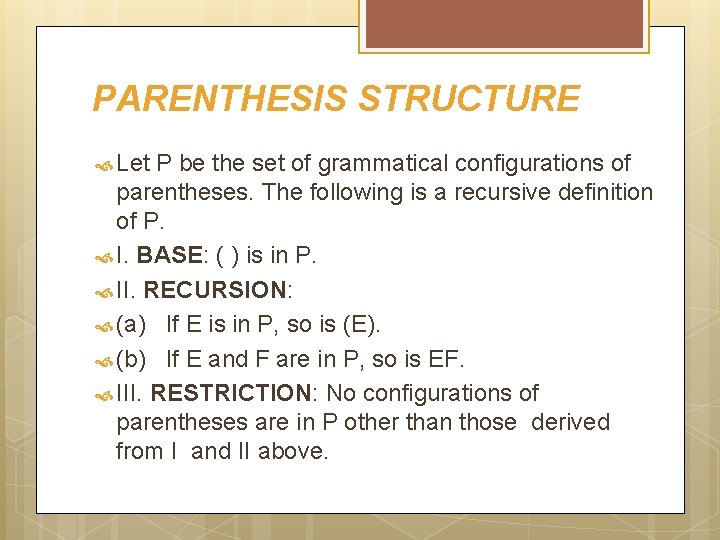PARENTHESIS STRUCTURE Let P be the set of grammatical configurations of parentheses. The following is a recursive definition of P. I. BASE: ( ) is in P. II. RECURSION: (a) If E is in P, so is (E). (b) If E and F are in P, so is EF. III. RESTRICTION: No configurations of parentheses are in P other than those derived from I and II above.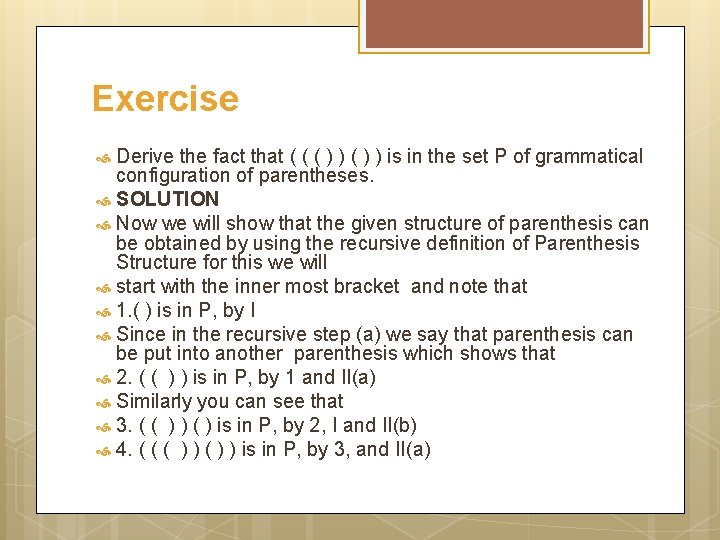Exercise Derive the fact that ( ( ( ) ) is in the set P of grammatical configuration of parentheses. SOLUTION Now we will show that the given structure of parenthesis can be obtained by using the recursive definition of Parenthesis Structure for this we will start with the inner most bracket and note that 1. ( ) is in P, by I Since in the recursive step (a) we say that parenthesis can be put into another parenthesis which shows that 2. ( ( ) ) is in P, by 1 and II(a) Similarly you can see that 3. ( ( ) ) ( ) is in P, by 2, I and II(b) 4. ( ( ( ) ) is in P, by 3, and II(a)SET OF ARITHMETIC EXPRESSIONS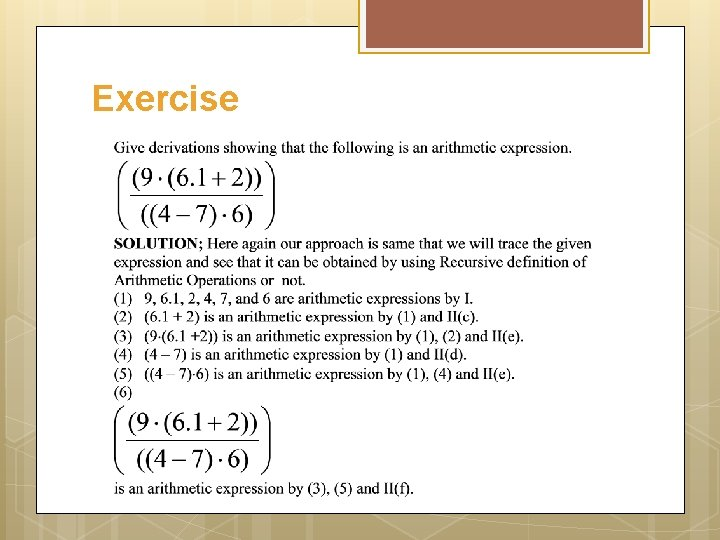Exercise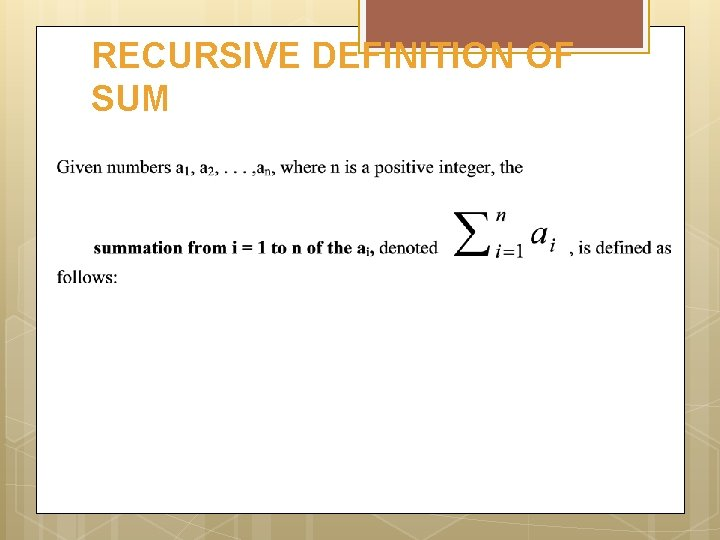RECURSIVE DEFINITION OF SUMExercise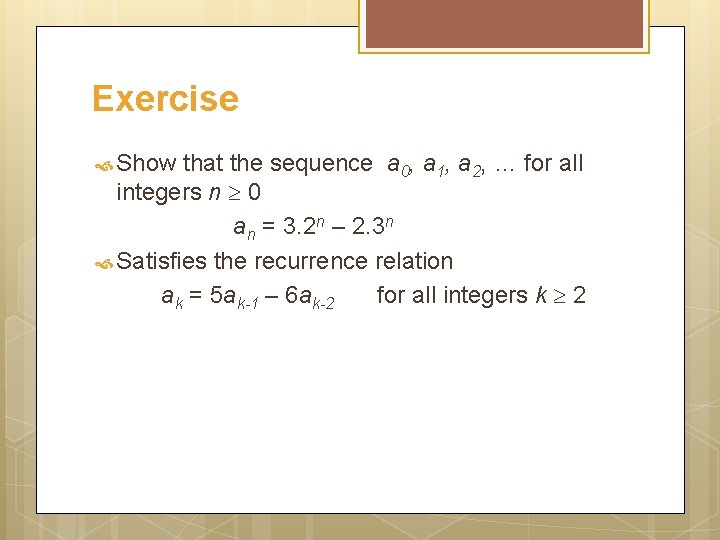Exercise Show that the sequence a 0, a 1, a 2, … for all integers n 0 an = 3. 2 n – 2. 3 n Satisfies the recurrence relation ak = 5 ak-1 – 6 ak-2 for all integers k 2